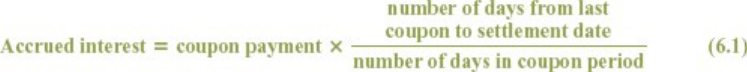Textbook Notes (270,000)
CA (160,000)
U of G (10,000)
ECON (700)
Chapter 6

# ECON 2560 Chapter Notes - Chapter 6: Accrued Interest, Current Yield, Interest Rate

Department
Economics
Course Code
ECON 2560
Professor
Nancy Bower
Chapter
6

This preview shows half of the first page. to view the full 3 pages of the document.Theory of Finance
Bonds
Chapter 6
Coupon:
The interest payment paid to the bondholder. 184
Face value:
Payment at the maturity of the bond. Also called par value or maturity value. 184
Coupon rate:
Annual interest payment as a percentage of face value. 184
Accrued interest:
Coupon interest earned from the last coupon payment to the purchase date of the bond. 186
Clean bond price:
Bond price excluding accrued interest. 186
Dirty bond price:
Bond price including accrued interest. 186
Annuity factors:
Present value of a \$1 received each of t periods, at various interest rates.
Discount factor or present value interest factor:
Present value of a \$1 future payment.
Current yield:
Annual coupon payment divided by current bond price. 191
Bond that sells for more than its face value. 191
Discount bond:
Bond that sells for less than its face value. 191
Yield to maturity:
Interest rate for which the present value of the bond's payments equals the price. 191
Interest Rates and Bond Prices (annually)